# CBSE Class 10 Maths Chapter 8-Introduction to Trigonometry Objective Questions

In this chapter 8- Introduction to Trigonometry from CBSE class 10 Maths, students are taught about the concept of trigonometry. This concept involves the study of some important ratios of the right angle triangle with respect to the angles called trigonometric ratios of the angles. From this chapter, students will also learn to establish some of the trigonometric identities and solve certain specific angles (from 0° to 90°) of the trigonometric ratios, sine, cosine, tangents and more. They can also learn other concepts like specific angles, ratios of complementary angles and so on. Here you are given access to the solutions of all the CBSE Class 10 Maths Chapter 8- Introduction to Trigonometry Objective Questions as well.

### List of Sub-Topics Discussed In Chapter 8

This comprises only the Multiple Choice Questions (MCQs) taken from various important topics in the chapter with a detailed solution and answers provided. The CBSE Class 10 Maths Objective Questions in the PDF are also arranged as per the topics given. Given that the changed exam pattern is expected to include more MCQs, these CBSE Class 10 Maths Chapter 8- Introduction to Trigonometry Objective Questions will help the students to prepare better for the exams.

8.1 Introduction (4 MCQs Are From This Topic)

8.2 Trigonometric Identities (4 MCQs From The Topic)

8.3 Trigonometric Ratios (4 MCQs From This Topic)

8.4 Trigonometric Ratios of Complementary Angles (4 MCQs Listed From The Topic)

8.5 Trigonometric Ratios of Specific Angles (4 MCQs From The Given Topic)

## Download CBSE Class 10 Maths Chapter 8-Introduction to Trigonometry Objective Questions Free PDF

### Introduction

1. In a right triangle ABC, the right angle is at B. Which of the following is true about the other two angles A and C?
1. There is no restriction on the measure of the angles
2. Both the angles should be obtuse
3. Both the angles should be acute
4. One of the angles is acute and the other is obtuse

Answer: (C) Both the angles should be acute

Solution: In triangle ABC, ∠A + ∠B + ∠C =180 °

∠A + ∠C= 180° – 90 ° = 90° ⇒None of the angles can be ≥ 90 °

1. In a right triangle ABC, the right angle is at B. What is the length of missing side in the figure?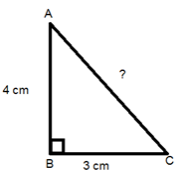1. 25 cm
2. 12cm
3. 7cm
4. 5cm

Solution: Pythagoras theorem: In a right angled triangle,

Hypotenuse2 = Sum of squares of other 2 sides

That is,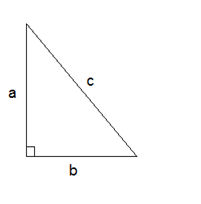c2=a2+b2

Here a = 4 cm and b = 3 cm,

So the missing side = c =

$$\begin{array}{l}\sqrt{3^{2}-4^{2}}\end{array}$$
= 5 cm

1. Which of the following numbers can form sides of a right angled triangle?
1. 13 cm , 27 cm , 15 cm
2. 4 cm , 5 cm , 9 cm
3. 2 cm , 17 cm , 9 cm
4. 10 cm , 6 cm , 8 cm

Answer: (D) 10 cm, 6 cm, 8 cm

Solution: The basic condition for any type of triangle is:

(i) The sum of 2 sides of a triangle should be greater than the third side

(ii) The difference of any 2 sides should be less than the third side.

For a triangle to be a right angled triangle, there is an additional condition.

Pythagoras theorem: In a right angled triangle,

Hypotenuse2= Sum of squares of other 2 sides

That is, c2=a2+b2; Also note that the hypotenuse is the largest side in a right triangle.

Considering each of the given options,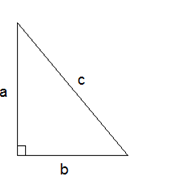102=62+82

172≠22+92

92≠52+42

272≠132+152

So, A is the correct option.

1. Which of the following are Pythagorean triplets?
1. 4 cm , 6 cm , 8 cm
2. 24 cm , 10 cm , 26 cm
3. 13 cm , 27 cm , 30 cm
4. 2 cm , 17 cm , 9 cm

Answer: (B) 24 cm, 10 cm, 26 cm

Solution: Pythagorean triplets are those set of numbers which satisfy the Pythagoras theorem.

Considering the options given to us –

82≠42+62

172≠22+92

262=242+102

302≠272+132

Therefore, 24, 10 and 26 are Pythagorean triplets.

### Trigonometric Identities

1. If secθ + tanθ = x, then tanθ is:
1. (x2-1) / 2x
2. (x2+1) / 2x
3. (x2-1) / x
4. (x2+1) / x

Solution: We know that, sec2θ – tan2θ = 1

Therefore, (secθ + tanθ) (secθ – tanθ) = 1

Since, (secθ + tanθ) = x

Thus, (secθ – tanθ) = 1/x

Solving both equations

We get tan θ = (x2-1) / 2x

1. If p cotθ =
$$\begin{array}{l}\sqrt{q^{2}-p^{2}}\end{array}$$
then the value of sinθ is ___. (θ being an acute angle)
1. q/3p
2. q/2p
3. p/q
4. 0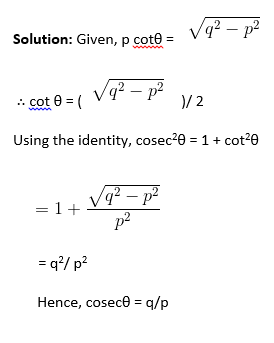∴ sin θ = p/q

1. If sin A = 8/17, find the value of secA cosA + cosecA cosA.
1. 23/8
2. 15/8
3. 8/15
4. 6/23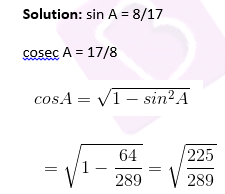= 15/17

sec A = 17/15

secA cosA + cosecA cosA = (17/15) * (15/17) + (17/15)  * (15/17)

= 1 + (15/8)

= 23/8

1. (sin A−2 sin3A)/ (2 cos3A−cos A)=
1. tan A
2. cot A
3. sec A
4. 1

Solutions: (sin A−2 sin3A)/ (2 cos3A−cos A) = (sin A (1−2 sin2A))/ (cos A(2 cos2A−1))

= (sin A (sin2A+cos2A−2 sin2A)) / (cos A (2 cos2A− (sin2A+cos2A))

= (sin A (cos2A−sin2A)) / (cos A (cos2A−sin2A))

=tan A

### Trigonometric Ratios

1. (cos A / cot A) + sin A= ____________
1. cot A
2. 2 sin A
3. 2 cos A
4. sec A

Solution: (cos A / cot A) + sin A

=Cos A / (cos A/sin A) + sin A

= sin A + sin A

= 2 sin A

1. If 5tanθ=4, then value of (5sinθ -4cosθ)/(5sinθ +4cosθ) is:
1. 1/6
2. 5/6
3. 0
4. 5/3

Solution: Divide both numerator and denominator by cos θ and solve

(5 sin θ- 4 cos θ)/ (5 sin θ + 4 cos θ)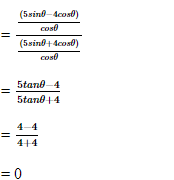(Since, given that 5 tan θ = 4 )

1. In △PQR, PQ = 12 cm and PR = 13 cm. ∠Q=90° Find tan P – cot R
1. –(119/60)
2. 119/60
3. 0
4. 1

Solution: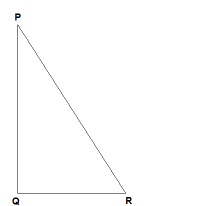Given that in △ PQR, PQ = 12 cm and PR = 13 cm.

Now, from Pythagoras theorem,

PQ2+QR2=PR2

⇒QR2=PR2−PQ2

⇒QR2=132−122

⇒QR2=169−144=25

⇒QR= √25 = 5 cm

Now, tan P= opposite side/ adjacent side = QR/PQ= 5/12

cot R= adjacent side/ opposite side = QR/PQ = 5/12

∴tan P−cot R= (5/12)-(5/12) = 0

1. If tanθ= (x sinϕ) / (1−xcosϕ) and, tan ϕ = (y sin θ)/ (1−y cos θ) then x/y =
1. sinθ / (1−cosϕ)
2. sinθ / (1−cosθ)
3. sinθ/sinϕ
4. sinϕ / sinθ

Solution: We have, tanθ = (x sinϕ)/ (1−xcosϕ)

⇒ (1−xcos ϕ) / (x sin ϕ) = 1/ tanθ ⇒ (1/ xsin ϕ) −cotϕ=cotθ

⇒ 1/ xsin ϕ= =cot θ+cot ϕ

and tan ϕ = y sinθ / (1−y cosθ) ⇒ (1−y cosθ)/ y sinθ = 1/ tan ϕ

⇒ (1/y sin θ) – cot θ = cotϕ⇒ (1/ y sin θ) =cot ϕ+cot θ

⇒ (1/y sin θ) = (1/ x sin ϕ) ⇒ x/y = sin θ/ sin ϕ

### Trigonometric Ratios of Complementary Angles

1. The value of tan1° × tan2° × tan3°  ×……× tan 89° is :
1. ½
2. 2
3. 1
4. 0

Solution: tanθcotθ=1,

tan (90−θ) =cotθ

and tan45°=1

Given: tan1°.tan2°,tan3° …….tan88°. tan89°

= (tan1°. tan89°),(tan2°. tan88°)…..(tan44°.tan46°) (tan45°)

= [(tan1°. tan (90°−1°)]. [(tan 2°. tan(90°−2°)]………. [(tan44°. tan(90°−44°)].1

= (tan1°. cot1°). (tan2°. cot2°) ……. (tan44°. cot44°)

= 1

1. If tan2A = cot(A-18°), then value of A is:
1. 27°
2. 24°
3. 36°
4. 18°

Solution: Given, tan 2A = cot (A – 18°)

⇒ tan 2A = tan (90 – (A – 18°)

⇒ tan 2A = tan (108° – A)

⇒ 2A = 108° – A

⇒ 3A = 108°

⇒ A = 36°

1. If tan 4θ=cot(θ−10°), where 4θ and(θ−10°) are acute angles then the value of θ in degrees is
1. 16°
2. 20°
3. 32°
4. 40°

Solution: Given, tan 4θ=cot(θ−10°)

This can be written as

cot(90°− 4θ)=cot(θ−10°) —–(i)

(∵ Tan θ = Cot(90°− θ))

Hence, from (i) we have

⇒90°− 4θ= θ−10°

⇒5θ =100°

⇒θ =20°

1. In the given triangle right angled at B, which pair of angles are complementary?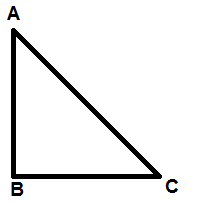1. None of these
2. C and A
3. A and B
4. B and C

Solution: Two angles are said to be complementary, if their sum is 90°. The triangle is right angled at B. With angle sum property of the triangle, ∠A+∠B+∠C=180°

∠A+∠C=90°, Hence angle A and C are complementary.

### Trigonometric Ratios of Specific Angles

1. Which of the following is correct for some θ, such that 0° ≤θ< 90°
1. 1/ cos θ < 1
2. sec θ = 0
3. 1/ sec θ < 1
4. 1/ sec θ> 1

Answer: (C) 1/ sec θ < 1

Solution: 1/ sec θ = cos θ. And value of cos θ ranges from 0 to 1

1. The value cot2 30°−2cos2 60°−3/4sec2 45°−4sin2 30° is
1. 2
2. -1
3. 1
4. 0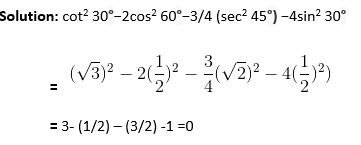1. If Cosec (A+ B) =
$$\begin{array}{l}\frac{2}{\sqrt{3}}\end{array}$$
sec(A-B)=
$$\begin{array}{l}\frac{2}{\sqrt{3}}\end{array}$$

0°<A+B≤90°,

Find A and B.

1. 25°,35°
2. 30°, 30°
3. 45°, 15°
4. 10°,50°

Solution: If A+B lies in this range 0°<A+B≤90°

cosec (A+B) =

$$\begin{array}{l}\frac{2}{\sqrt{3}}\end{array}$$
only when A+B=60° …….. (1)

sec (A-B) =

$$\begin{array}{l}\frac{2}{\sqrt{3}}\end{array}$$
only when A−B=30° ……..(2)

By Solving equation 1 and equation 2

A=45° and B=15°

1. cos 1° × cos 2° × cos 3° ×……..× cos 180° is equal to:
1. 0
2. 1
3. ½
4. -1

Solution: Since cos 90° = 0

The given expression

cos 1° × cos 2° × cos 3° ×….× cos 90° ×……..× cos 180°

reduces to zero as it contains cos 90° which is equal to 0

Students can practice these multiple choice questions in order to self- assess their knowledge of the important topics discussed in this chapter. They can download the free PDF of the objective questions from the link given above.

The solutions for these MCQs are also given in step-by-step procedure for the students to refer. However, students preparing for CBSE Class 10 Maths Board Examination are advised to practice the given question for Mathematics on their own without referring to the answers or solutions, in order to self- assess their performance. Find also some CBSE Class 10 Maths Chapter 8 Extra MCQs.

## CBSE Class 10 Maths Chapter 8 Extra MCQs

1.  Given that cos A = 4/5, then calculate tan A.
(A) 3/5
(B) 3/4
(C) 4/3
(D) 4/5

2. (sin30° + cos30°) – (sin 60° + cos60°)
(A) – 1
(B) 0
(C) 1
(D) 2

All that students wish to know about the CBSE Class 10 and its study resources is available here at BYJU’S. Stay tuned for more updates.